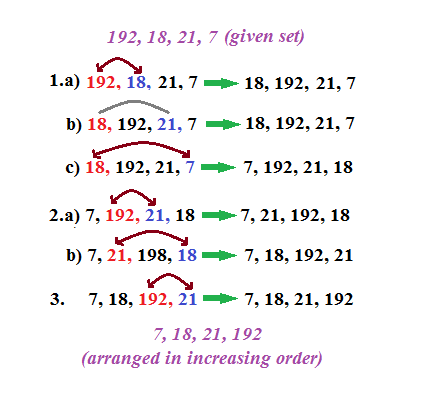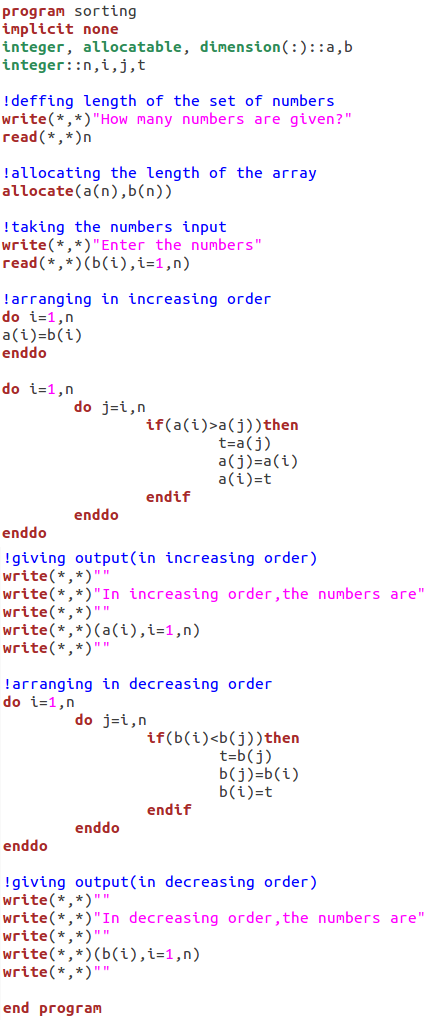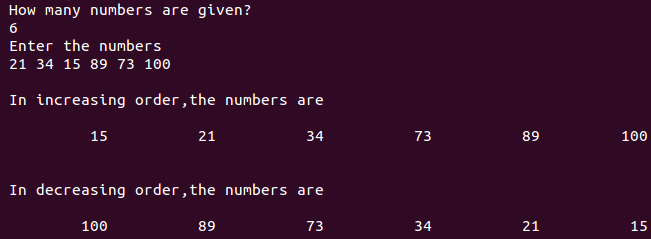# Sorting Some Numbers in a Certain Order (Increasing or Decreasing): A Fortran90 Code

Knoji reviews products and up-and-coming brands we think you'll love. In certain cases, we may receive a commission from brands mentioned in our guides. Learn more.
Sorting of numbers in increasing or decreasing order is not a very hard work until we have a lot of numbers to be sorted. But if we have such a job, we look for some programming which can make our job easy. Here I am going to describe how to write a fortr

We often need to arrange some given numbers in increasing or decreasing order. This job does not seem to be very tough until the numbers are a lot in number. But when we have to sort a lot of numbers, then it may not be so easy and be a time wasting job. Program can make this work very easy. Here we will learn how to sort some real numbers using Fortran90, an easy and well known programming language.

Algorithm of sorting some real numbers

We will discuss the algorithm of arranging some real numbers in increasing order because arranging them in decreasing order is a similar job. Let us do this for 4 numbers and the numbers are given like “192, 18, 21, 7”. Steps are as following:

1. a) We will start with the first number (here 192) and check if it is greater or less than the second one (here 18).

i) If the first one is less than the second number, we have to do nothing.

ii)  If the first one is greater (as here 192>18), we have to swap them i.e. first number will be on the second position and the second number will go to the first position. Then the set will become “18, 192, 21, 7”

b) We will now pick up the new first number (i.e. 18) and compare it with third number (i.e. 21). Step (1.a.i) and (1.a.ii) is same in this step too. As 18

At the end of this step the set will become “18, 192, 21, 7”

c) Now it is the time to compare the first number with the forth number as 18<7,we have to follow (1.a.ii). So our set is now “7, 192, 21, 18”.

So we see that step (1) has dragged the lowest number to the first position. Now we have to worry about the rest 3 number. To do this we have to pick up the second number (i.e. 192) and do the exactly same job as (1.a), (1.b), (1.c).

2. a) As 192>21, they will swap and make the set as “7, 21, 192, 18”

b) As 21>18, they will swap also. Now we get “7, 18, 192, 21”

3) We are now left with the last two numbers disordered. As 192>21, we will swap them and get a properly arranged set of numbers (in increasing order) as “7, 18, 21, 192”.

A pictorial representation makes the process easy to understand.Programming

Let us now try to write a fortran90 program to sort a set of numbers in increasing order. I am giving the code for arranging them in decreasing order too in the same program to help the readers understand the logic clearly though we can just print the numbers, arranged in increasing order, reversely to get them in decreasing order. Again we will use dynamic memory allocation to make our program general and be able to use it for any arbitrary length of the given set of numbers.A typical output is given belowSo we see sorting some given numbers in desired order using Fortran90 is not a very much difficult job.